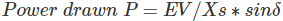# MCQs on Electrical Machines

##### Page 48 of 114. Go to page 1 2 3 4 5 6 7 8 9 10 11 12 13 14 15 16 17 18 19 20 21 22 23 24 25 26 27 28 29 30 31 32 33 34 35 36 37 38 39 40 41 42 43 44 45 46 47 48 49 50 51 52 53 54 55 56 57 58 59 60 61 62 63 64 65 66 67 68 69 70 71 72 73 74 75 76 77 78 79 80 81 82 83 84 85 86 87 88 89 90 91 92 93 94 95 96 97 98 99 100 101 102 103 104 105 106 107 108 109 110 111 112 113 114
01․ The leakage reactance of a 3-phase alternator is determined by performing
open circuit and zero power factor tests.
zero power factor and slip tests.
open circuit and short circuit tests.
short circuit and slip tests.

In synchronous impedance method voltage regulation is determined by calculating synchronous impedance Zs. MMF method also deals with determining voltage drop through MMF, but the drops in machine 'Ia*Xl' is a voltage quantity and 'Ia*Xar' is a MMF quantity as armature reaction is the reduction of flux which is represented through voltage drop. In potier method voltage quantities are calculated on voltage basis and MMF quantities are calculated on MMF basis. The method also separates leakage reactance and armature reactance and is known as potier reactance. It requires open circuit, short circuit and zero power factor tests. But to find only leakage reactance open circuit and zero power factor tests are sufficient.

02․ Slip test is performed to determine
slip
direct axis reactance and quadrature axis reactance
positive sequence reactance and negative sequence reactance
all of the above

In salient pole machine air gap between the stator and rotor is non uniform. To find the voltage regulation of a salient pole machine it requires direct axis reactance and quadrature axis reactance. These two values are determined by conducting slip test on a given salient pole machine, Which is run at a speed slightly less than its synchronous speed. Direct axis reactance Xd = Vmax/Imin Quadrature axis reactance Xq = Vmin/Imax

03․ The results of a slip test for determining direct axis (Xd) and quadrature axis (Xq) reactances of a star connected salient pole alternator are given below Phase values: Vmax = 108 V ; Vmin = 96 V Imax = 12 A ; Imin = 10 A Hence the two reactances will be
Xd = 10.8Ω and Xq = 8Ω
Xd = 9Ω and Xq = 9.6Ω
Xd = 9.6Ω and Xq = 9Ω
Xd = 8Ω and Xq = 10.8Ω

Direct axis reactance Xd = Vmax/Imin = 108/10 = 10.8Ω Quadrature axis reactance Xq = Vmin/Imax = 96/12 = 8Ω

04․ Number of slip rings in 3-phase synchronous motor will be
0
1
2
3 or 4

In 3-phase synchronous motor, armature is stationary and poles are rotating. 3-phase AC supply is given to stator and DC excitation is given to rotor in this machine. To give DC excitation to the rotor two slip rings are required.

05․ In a synchronous motor torque or load angle δ ------------- with increase in load
increases
decreases
remains unaffected
none of the above

Power P = EV/Xs * sinδ P ∝ sinδ As load increases, power drawn by the motor will increase. Therefore load angle or torque angle δ also increases.

06․ Synchronising power of a synchronous motor varies
directly as synchronous reactances Xs
directly as Xs2
inversely as 1/Xs
all of the above

Synchronising power P = EV/Xs * sinδ Synchronising power P ∝ 1/Xs Therefore, synchronising power of a synchronous motor varies inversely as 1/Xs.

07․ For a 3-phase, 4 pole, 50 Hz synchronous motor the frequency, pole number and the frequency are halved. The motor speed will be
375 rpm
75 rpm
1500 rpm
3000 rpm

Synchronous motor will run at synchronous speed Ns. Synchronous speed Ns = 120 *f/P If pole number and frequency are halved, Synchronous speed Ns = 120 *(f/2)/(P/2) = 120 * f/P Ns = 120 * 50 /4 = 1500 rpm

08․ Maximum power developed by a synchronous motor depends on
supply voltage and excitation
supply voltage, load angle and excitation

Power developed P = EV/Xs * sinδ Where, E = Excitation V = Supply voltage Xs = Synchronous reactance δ = Load angle Maximum power will developed when δ = 90° Pmax = EV/Xs Therefore Maximum power is independent of load angle.

09․ Stability of a synchronous motor ---------- with the increase in excitation.
increases
decreases
remains unaffected
any of the aboveFor constant load as excitation E increases, the load angle δ will decrease. Therefore stability will increase. If the load angle is maximum, stability is minimum and vice versa. Stability limit is 90o.

10․ Which of the following motor has more stability
cylindrical motor
salient pole motor
both motors has same stability
none of the above

In cylindrical motor maximum power is drawn at 90°. But in salient pole motor maximum power is drawn at below 90° due to reluctance power. Therefore salient mole machine has more stability than cylindrical motor.

<<<4647484950>>>